# Chapter 7 Fluid Dynamics and Hyperbolic Equations

## 7.1  The fluid momentum equation

identifies all the sources of momentum within a particular volume $V$ and the fluxes of momentum inward across the boundary $\partial V$ of that volume, and sets their sum equal to the rate of change of the total momentum in the volume. Momentum is of course a vector quantity whose density (momentum per unit volume) is $\mathit{\rho }\mathbit{v}$. The total rate of change of momentum is the integral of this quantity over the volume.
The sources of momentum within a volume consist of any body forces that might be acting upon the fluid. This, of course, is what Newton's second law of motion tells us. Rate of change of momentum is equal to force. However, like the momentum, the force must be expressed in terms of force density, $\mathbit{F}$, the force per unit volume acting on the fluid. For example, gravity gives rise to a force per unit volume $\mathit{\rho }\mathbit{g}$, where $\mathbit{g}$ is the gravitational acceleration vector (downwards on earth). Or again, if the fluid is electrically charged with a charge density ${\mathit{\rho }}_{q}$, then the body force density arising from an electric field $\mathbit{E}$ is ${\mathit{\rho }}_{q}\mathbit{E}$. The force density $\mathbit{F}$ is the sum of all such forces that happen to be present. There might be none.
The flux of momentum across the surface is the more tricky part. Some of that flux arises because of fluid motion. The fluid momentum, density $\mathit{\rho }\mathbit{v}$, is being carried along, "convected", with the fluid at velocity $\mathbit{v}$. Consequently, across any stationary surface element $\mathbit{dA}$ there is a convective flux of momentum equal to $\mathit{\rho }\mathbit{v}\mathbit{v}.\mathbit{dA}$. We may therefore identify the convective momentum flux density as the quantity whose dot product with $\mathbit{dA}$ gives the flux across $\mathbit{dA}$. It is $\mathit{\rho }\mathbit{v}\mathbit{v}$, which is a tensor (or dyadic in this notation), it has two sets of coordinate indices, and can be thought of as a 3$×$3 matrix:
 $\mathit{\rho }\mathbit{v}\mathbit{v}=\mathit{\rho }{v}_{i}{v}_{j}=\mathit{\rho }\left(\begin{array}{ccc}\hfill {v}_{1}{v}_{1}\hfill & \hfill {v}_{1}{v}_{2}\hfill & \hfill {v}_{1}{v}_{3}\hfill \\ \hfill {v}_{2}{v}_{1}\hfill & \hfill {v}_{2}{v}_{2}\hfill & \hfill {v}_{2}{v}_{3}\hfill \\ \hfill {v}_{3}{v}_{1}\hfill & \hfill {v}_{3}{v}_{2}\hfill & \hfill {v}_{3}{v}_{3}\hfill \end{array}\right).$ $\left(7.1\right)$
In addition to this convective momentum flux, carried by the local mean fluid velocity, there may be momentum flux that arises from other effects. One such effect is pressure. Another is viscosity. Another (in non-Newtonian fluids like gels or of course solids) might be shear stress arising from elasticity. All of these can be lumped together into another tensor that is usually called simply the stress tensor, or the pressure tensor. We'll write it $\mathbf{P}$. It is a 3$×$3 matrix with coefficients ${P}_{\mathit{ij}}$. We assume that just as $\mathbit{F}$ is the sum of all possible body force densities, $\mathbf{P}$ is the sum of all non-convective momentum flux densities.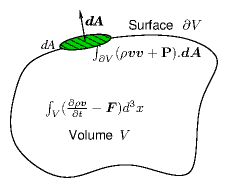Figure 7.1: Integral of momentum flux density across the boundary surface $\partial V$ is equal to minus the integral of rate of change of momentum minus force density over the volume $V$. The momentum flux density includes convective flux and stress tensor parts.
The conservation of momentum is then
 $\frac{\partial }{\partial t}{\int }_{V}\mathit{\rho }\mathbit{v}{d}^{3}x={\int }_{V}\mathbit{F}{d}^{3}x-{\int }_{\partial V}\left(\mathit{\rho }\mathbit{v}\mathbit{v}+\mathbf{P}\right).\mathbit{dA}.$ $\left(7.2\right)$
applied to an arbitrary volume and surface, as illustrated in Fig. 7.1. Just as with mass conservation, we can use Gauss's divergence theorem to turn the surface integral into a volume integral, and gather the terms together:
 ${\int }_{V}\frac{\partial }{\partial t}\left(\mathit{\rho }\mathbit{v}\right)-\mathbit{F}+\nabla .\left(\mathit{\rho }\mathbit{v}\mathbit{v}+\mathbf{P}\right){d}^{3}x=0.$ $\left(7.3\right)$
This equation must hold for any volume $V$, and the only way for that to be true is for the integrand to be zero everywhere:
 $\frac{\partial }{\partial t}\left(\mathit{\rho }\mathbit{v}\right)-\mathbit{F}+\nabla .\left(\mathit{\rho }\mathbit{v}\mathbit{v}+\mathbf{P}\right)=0.$ $\left(7.4\right)$
This is the general form of the fluid momentum conservation equation. If we know what $\mathbf{P}$ is, then this equation is enough to solve for $\mathbit{v}$. But really we are in the same situation as we were with the continuity equation. There, we could solve the equation for $\mathit{\rho }$, but only if we knew $\mathbit{v}$. Now we've got an equation for $\mathbit{v}$, but it depends upon knowing $\mathbf{P}$. Intuitively you can see that this heirarchical process might go on for ever. We can get an equation for $\mathbf{P}$ from the conservation of energy, but that equation will contain a third-order tensor governing the energy flux (conduction etc.). Solving for that requires yet another equation, and so on. In general, to get a soluble problem we have to call a halt at some point - a process called "closure". How and when we do that decides what sort of fluid equations we end up with. This closure generally invokes a "constitutive relation" between a property such as stress and the other variables of the fluid such as density or velocity gradient.
The kinds of fluids we encounter most in everyday life are isotropic. They have no intrinsically preferred direction. There are fluids that are anisotropic, for example plasmas or other electrically conducting fluids in magnetic fields. But for now we set them aside. Isotropic fluids generally give rise to nearly symmetric stress tensors $\mathbf{P}$. It is then helpful to separate out the total stress tensor into a part that is simply a scalar $p$ times the unit matrix $\mathbf{I}$ (that's the isotropic part), and a part $\mathbit{\sigma }$ that has zero trace, i.e. the sum of its diagonal elements is zero $\sum _{i}{\mathit{\sigma }}_{\mathit{ii}}=0$. Thus we write $\mathbf{P}=p\mathbf{I}+\mathbit{\sigma }$. Then $p$ is the pressure.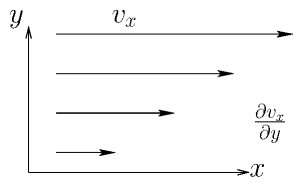Figure 7.2: The transfer in the $y$-direction of $x$-momentum arises from the rate of strain ${\mathit{dv}}_{x}/\mathit{dy}$. The rate of strain tensor is the symmetric generalization of this form.
The traceless stress tensor $\mathbit{\sigma }$ for simple fluids arises from viscosity, which relates stress to the rate of strain tensor, see Fig. 7.2. The rate of strain tensor is $\frac{1}{2}\left(\frac{\partial {v}_{i}}{\partial {x}_{j}}+\frac{\partial {v}_{j}}{\partial {x}_{i}}\right)$. And $\mathbit{\sigma }$ is proportional to its traceless part
 ${\mathit{\sigma }}_{\mathit{ij}}=\mathit{\mu }\left[\left(\frac{\partial {v}_{i}}{\partial {x}_{j}}+\frac{\partial {v}_{j}}{\partial {x}_{i}}\right)-\frac{2}{3}\nabla .\mathbit{v}{\mathit{\delta }}_{\mathit{ij}}\right]=\mathit{\mu }{\left[\left(\left(\nabla \mathbit{v}\right)+\left(\nabla \mathbit{v}{\right)}^{T}\right)-\frac{2}{3}\left(\nabla .\mathbit{v}\right)\mathbf{I}\right]}_{\mathit{ij}}.$ $\left(7.5\right)$
The constant of proportionality, $\mathit{\mu }$ is the (shear) viscosity. Here $\left(\nabla \mathbit{v}\right)$ is a tensor (dyadic) whose transpose is indicated with a superscript $T$. Substituting this expression into the general momentum conservation equation gives what is called the Navier-Stokes equation.
 $\frac{\partial }{\partial t}\left(\mathit{\rho }\mathbit{v}\right)+\nabla .\left(\mathit{\rho }\mathbit{v}\mathbit{v}\right)=-\nabla .\left(p\mathbf{I}+\mathbit{\sigma }\right)+\mathbit{F}=-\nabla p-\mathit{\mu }{\nabla }^{2}\mathbit{v}-\frac{1}{3}\mathit{\mu }\nabla \left(\nabla .\mathbit{v}\right)+\mathbit{F}.$ $\left(7.6\right)$
The closure for the pressure (and viscosity) must generally be determined by equations of state relating pressure, $p$, to density, $\mathit{\rho }$. For example for an ideal isothermal gas $p\propto \mathit{\rho }$. Liquids have an equation of state that amounts approximately to incompressibility, $\mathit{\rho }=\mathit{const}.$, and they generally have zero volumetric source $S$. For such a fluid, the continuity equation shows that the velocity divergence is zero, $\nabla .\mathbit{v}=0$. The divergenceless fluid momentum equation is then simpler.
 $\frac{\partial }{\partial t}\left(\mathit{\rho }\mathbit{v}\right)+\nabla .\left(\mathit{\rho }\mathbit{v}\mathbit{v}\right)=-\nabla p-\mathit{\mu }{\nabla }^{2}\mathbit{v}+\mathbit{F}.$ $\left(7.7\right)$
And of course if viscosity and body forces are negligible it becomes even simpler yet.
The left hand side of these equations is often rewritten using the continuity equation with $S=0$ to demonstrate
 $\frac{\partial }{\partial t}\left(\mathit{\rho }\mathbit{v}\right)+\nabla .\left(\mathit{\rho }\mathbit{v}\mathbit{v}\right)=\mathit{\rho }\left(\frac{\partial }{\partial t}\mathbit{v}+\mathbit{v}.\nabla \mathbit{v}\right).$ $\left(7.8\right)$
Then the second form is recognized as $\mathit{\rho }$ times the convective derivative $\partial /\partial t+\mathbit{v}.\nabla$ of $\mathbit{v}$. However, the first form is what is called "conservative" form, and it is by far the better form to use for discrete representation and numerical solution on fixed meshes.

## 7.2  Hyperbolic Equations

Fluid equations are generally hyperbolic. Let's start our analysis of such hyperbolic equations by considering a problem where body force is zero; sources are zero; viscosity is zero; pressure is related to density by an adiabatic law $p{\mathit{\rho }}^{-\mathit{\gamma }}=\mathit{const}.$; and the configuration is one-dimensional in space. This is governed then by the following equations.
 $\begin{array}{cccc}\mathit{Continuity}:\mathrm{ }\hfill & \hfill \frac{\partial \mathit{\rho }}{\partial t}+\frac{\partial }{\partial x}\left(\mathit{\rho }v\right)& \hfill =\hfill & 0\hfill \\ \mathit{Momentum}:\mathrm{ }\hfill & \hfill \frac{\partial }{\partial t}\left(\mathit{\rho }v\right)+\frac{\partial }{\partial x}\left(\mathit{\rho }{v}^{2}\right)& \hfill =\hfill & -\frac{\partial }{\partial x}p\hfill \\ \mathit{State}:\mathrm{ }\hfill & \hfill p{\mathit{\rho }}^{-\mathit{\gamma }}& \hfill =\hfill & \mathit{const}.\hfill \end{array}$ $\left(7.9\right)$
These are three equations for three unknowns $\mathit{\rho }$, $v$, and $p$. They represent a compressible fluid (gas) in a pipe, for example. We can eliminate $p$ immediately by writing $p={p}_{0}{\mathit{\rho }}^{\mathit{\gamma }}/{\mathit{\rho }}_{0}^{\mathit{\gamma }}$. To retain the conservation properties, we regard the density, $\mathit{\rho }$, and momentum density, $\mathit{\rho }v=\mathit{\Gamma }$, as the independent variables, in which case the equations become
 $\begin{array}{ccc}\hfill \frac{\partial \mathit{\rho }}{\partial t}& \hfill =\hfill & -\frac{\partial \mathit{\Gamma }}{\partial x}\hfill \\ \hfill \frac{\partial \mathit{\Gamma }}{\partial t}& \hfill =\hfill & -\frac{\partial }{\partial x}\left({\mathit{\Gamma }}^{2}/\mathit{\rho }+\left({p}_{0}/{\mathit{\rho }}_{0}^{\mathit{\gamma }}\right){\mathit{\rho }}^{\mathit{\gamma }}\right)\hfill \end{array}.$ $\left(7.10\right)$
We might well want to solve these nonlinear equations numerically. They are now expressed in a form that is actually the same for all types of fluid conservation equations:
 $\frac{\partial \mathbf{u}}{\partial t}=-\frac{\partial \mathbf{f}}{\partial x}.$ $\left(7.11\right)$
In our particular case
 $\mathbf{u}=\left(\begin{array}{c}\hfill \mathit{\rho }\hfill \\ \hfill \mathit{\Gamma }\hfill \end{array}\right),\mathrm{ }\text{and}\mathrm{ }\mathbf{f}=\left(\begin{array}{c}\hfill \mathit{\Gamma }\hfill \\ \hfill {\mathit{\Gamma }}^{2}/\mathit{\rho }+\left({p}_{0}/{\mathit{\rho }}_{0}^{\mathit{\gamma }}\right){\mathit{\rho }}^{\mathit{\gamma }}\hfill \end{array}\right)$ $\left(7.12\right)$
are the state vector and the flux vector respectively. Since the flux vector is a function of the state vector, we can use the chain rule to write the equations as
 $\frac{\partial \mathbf{u}}{\partial t}=-\frac{\partial \mathbf{f}}{\partial \mathbf{u}}\frac{\partial \mathbf{u}}{\partial x}=-\sum _{m=1,M}\frac{\partial \mathbf{f}}{\partial {u}_{m}}\frac{\partial {u}_{m}}{\partial x}=-\mathbf{J}\frac{\partial \mathbf{u}}{\partial x}.$ $\left(7.13\right)$
Here, the Jacobian matrix $\mathbf{J}=\partial \mathbf{f}/\partial \mathbf{u}$ has size $M×M=2×2$ and is explicitly
 $\mathbf{J}=\left(\begin{array}{cc}\hfill 0\hfill & \hfill 1\hfill \\ \hfill -{\mathit{\Gamma }}^{2}/{\mathit{\rho }}^{2}+\mathit{\gamma }\left({p}_{0}/{\mathit{\rho }}_{0}^{\mathit{\gamma }}\right){\mathit{\rho }}^{\mathit{\gamma }-1}\hfill & \hfill 2\mathit{\Gamma }/\mathit{\rho }\hfill \end{array}\right).$ $\left(7.14\right)$
The Jacobian matrix quite generally embodies the differential equation by relating time-derivates to space-derivatives of the state vector, through eq. (7.13): $\frac{\partial \mathbf{u}}{\partial t}=-\mathbf{J}\frac{\partial \mathbf{u}}{\partial x}$.
Writing for convenience $\mathit{\Gamma }/\mathit{\rho }=v$, and $\mathit{\gamma }\left({p}_{0}/{\mathit{\rho }}_{0}^{\mathit{\gamma }}\right){\mathit{\rho }}^{\mathit{\gamma }-1}={c}_{s}^{2}$, the eigenvalues of $\mathbf{J}$ are then solutions of
 $|\begin{array}{cc}\hfill -\mathit{\lambda }\hfill & \hfill 1\hfill \\ \hfill -{v}^{2}+{c}_{s}^{2}\hfill & \hfill -\mathit{\lambda }+2v\hfill \end{array}|={\mathit{\lambda }}^{2}-2v\mathit{\lambda }+{v}^{2}-{c}_{s}^{2}=0,$ $\left(7.15\right)$
which are
 $\mathit{\lambda }=v±{c}_{s}.$ $\left(7.16\right)$
For small density perturbations, ${c}_{s}^{2}=\mathit{\gamma }\left({p}_{0}/{\mathit{\rho }}_{0}^{\mathit{\gamma }}\right){\mathit{\rho }}^{\mathit{\gamma }-1}\approx \mathit{\gamma }{p}_{0}/{\mathit{\rho }}_{0}$, which gives the usual definition of the (small-amplitude) sound speed ${c}_{s}=\sqrt{\mathit{\gamma }{p}_{0}/{\mathit{\rho }}_{0}}$.
The fact that the eigenvalues are real is a demonstration that the system of equations is hyperbolic. The eigenvalues indicate the speed of propagation of disturbances. In this fluid they propagate at the speed of sound measured in the rest-frame of the fluid.

## 7.3  Finite Differences and Stability

Now let's consider possible finite difference representations of the equations. We notice that the simplest time differences give the time derivative $\left({\mathbf{u}}_{j}^{\left(n+1\right)}-{\mathbf{u}}_{j}^{\left(n\right)}\right)/\mathit{\Delta }t$ effectively at time $n+1/2$ but position $j$, and the simplest space difference gives a derivative $\left({\mathbf{f}}_{j+1}^{\left(n\right)}-{\mathbf{f}}_{j}^{\left(n\right)}\right)/\mathit{\Delta }x$ at position $j+1/2$. These expressions don't line up with one another so if we use them, we'll certainly have only first order accuracy. See Fig. 7.3.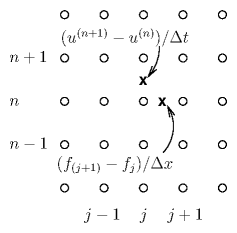Figure 7.3: Derivatives in time ($n$) and space ($j$) implemented as finite differences give rise to values at the half-mesh points x.
We could try to fix that by taking centered derivatives; but it turns out that may make things worse. The scheme may become unstable. But how do we analyse stability for this fluid? We have multiple coupled dependent variables. How do we deal with that? The answer, in summary, is that we find the combinations of dependent variables that behave in a way that is approximately uncoupled from the other combinations of dependent variables - in other words, the characteristic modes of the system. Then we analyse the Von Neumann stability of those modes separately.
If the Jacobian matrix is independent of position, then it is possible to change the dependent variables to new combinations of variables, each of which is uncoupled from the other combinations${}^{42}$. The new combination to use consists of the eigenvectors of the matrix $\mathbf{J}$.
Let's illustrate this with our fluid. Consider the eigenvalues $\mathit{\lambda }=v±{c}_{s}$. For each eigenvalue, the eigenvector, which is the solution of the homogeneous equation $\left(\mathbf{J}-\mathit{\lambda }\mathbf{I}\right)\mathbf{e}=0$, must give zero when multiplied by any of the rows of the combined matrix $\left(\mathbf{J}-\mathit{\lambda }\mathbf{I}\right)$. Using the top row, which becomes $\left(-\left[v±{c}_{s}\right],1\right)$ we find that the eigenvector is proportional to
 ${\mathbf{e}}_{±}=\left(\begin{array}{c}\hfill 1\hfill \\ \hfill v±{c}_{s}\hfill \end{array}\right)$ $\left(7.17\right)$
Now we can express any vector state as the sum of two coefficients ${q}_{±}$ times the two eigenvectors${}^{43}$ $\mathbf{u}={q}_{+}{\mathbf{e}}_{+}+{q}_{-}{\mathbf{e}}_{-}$ or written out
 $\left(\begin{array}{c}\hfill \mathit{\rho }\hfill \\ \hfill \mathit{\Gamma }\hfill \end{array}\right)={q}_{+}\left(\begin{array}{c}\hfill 1\hfill \\ \hfill v+{c}_{s}\hfill \end{array}\right)+{q}_{-}\left(\begin{array}{c}\hfill 1\hfill \\ \hfill v-{c}_{s}\hfill \end{array}\right).$ $\left(7.18\right)$
[The coefficient values are ${q}_{±}=\left[\mathit{\rho }\left(v\mp {c}_{s}\right)-\mathit{\Gamma }\right]/\left(±2{c}_{s}\right)$ but we don't need to know that.] The quantities ${q}_{±}$ can be considered to be the coefficients of the new vector representation $\mathbf{q}=\left(\genfrac{}{}{0}{}{{q}_{+}}{{q}_{-}}\right)$, by which the state can be expressed. Then the result of multiplying $\mathbf{u}$ by the Jacobian matrix can be written in terms of the new set of $q$-coefficients as follows,
 $\mathbf{J}\mathbf{u}={q}_{+}\mathbf{J}{\mathbf{e}}_{+}+{q}_{-}\mathbf{J}{\mathbf{e}}_{-}={q}_{+}{\mathit{\lambda }}_{+}{\mathbf{e}}_{+}+{q}_{-}{\mathit{\lambda }}_{-}{\mathbf{e}}_{-}\mathrm{ }.$ $\left(7.19\right)$
This shows that the vector of eigenvalue coefficients giving $\mathbf{J}\mathbf{u}$ is $\left(\genfrac{}{}{0}{}{{q}_{+}{\mathit{\lambda }}_{+}}{{q}_{-}{\mathit{\lambda }}_{-}}\right)$. So in terms of the new $q$-representation
 $\stackrel{‾}{\mathbf{J}}\mathbf{q}=\left(\genfrac{}{}{0}{}{{q}_{+}{\mathit{\lambda }}_{+}}{{q}_{-}{\mathit{\lambda }}_{-}}\right)=\left(\begin{array}{cc}\hfill {\mathit{\lambda }}_{+}\hfill & \hfill 0\hfill \\ \hfill 0\hfill & \hfill {\mathit{\lambda }}_{-}\hfill \end{array}\right)\left(\begin{array}{c}\hfill {q}_{+}\hfill \\ \hfill {q}_{-}\hfill \end{array}\right).$ $\left(7.20\right)$
In this $q$-representation, the operator $\mathbf{J}$, is represented by a different matrix $\stackrel{‾}{\mathbf{J}}$ which is diagonal having coefficients equal to the eigenvalues. Consequently the equations governing the evolution of the coefficients $\mathbf{q}$ of the eigenvectors separates into two independent equations
 $\frac{\partial {q}_{±}}{\partial t}=-{\mathit{\lambda }}_{±}\frac{\partial {q}_{±}}{\partial x},$ $\left(7.21\right)$
in place of the previously coupled equations governing $\mathbf{u}$. This process is totally general and will work for vectors of any dimensionality, corresponding to any order differential equations. We can now analyse each scalar equation separately for stability. Recognize, though, that the eigenvalues are not necessarily uniform in space, therefore this separation of the equations applies really only locally. So the stability analysis we now do is an approximate local analysis, not a precise global analysis.

### 7.3.1  FTCS is unstable

A forward time centered space difference scheme might spring to mind as a natural one, illustrated in Fig. 7.4.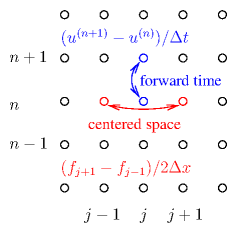Figure 7.4: Forward derivative in Time ($n$) and Centered in Space ($j$) (FTCS) finite differences give rise to an unstable scheme for hyperbolic problems.
For stability analysis purposes, we can suppose that we are using the new representation (in other words $u$ stands for each $q$ which we can consider separately in scalar equations). But we don't actually do the transformation to that new representation when implementing the scheme (only when analysing its stability). The first time through we'll do things explicitly but then take short cuts thereafter not bringing $q$ in explicitly. We write out the difference equation as
 ${\mathbf{u}}_{j}^{\left(n+1\right)}-{\mathbf{u}}_{j}^{\left(n\right)}=-\frac{\mathit{\Delta }t}{2\mathit{\Delta }x}\left({\mathbf{f}}_{j+1}^{\left(n\right)}-{\mathbf{f}}_{j-1}^{\left(n\right)}\right)=-\frac{\mathit{\Delta }t}{2\mathit{\Delta }x}\mathbf{J}\left({\mathbf{u}}_{j+1}^{\left(n\right)}-{\mathbf{u}}_{j-1}^{\left(n\right)}\right),$ $\left(7.22\right)$
which in the new representation is
 ${q}_{j}^{\left(n+1\right)}-{q}_{j}^{\left(n\right)}=-\frac{\mathit{\Delta }t}{2\mathit{\Delta }x}\mathit{\lambda }\left({q}_{j+1}^{\left(n\right)}-{q}_{j-1}^{\left(n\right)}\right)$ $\left(7.23\right)$
and consider a single spatial Fourier mode of $u$ and $f$ and hence of $q$
 ${q}_{j}=q\mathrm{exp}\left({\mathit{ik}}_{x}j\mathit{\Delta }x\right),\mathrm{ }{f}_{j}=f\mathrm{exp}\left({\mathit{ik}}_{x}j\mathit{\Delta }x\right).$ $\left(7.24\right)$
Substituting for the spatial dependence, the advancing equation becomes
 ${q}_{j}^{\left(n+1\right)}=\left[1-\frac{\mathit{\Delta }t\mathit{\lambda }}{2\mathit{\Delta }x}\left({e}^{{\mathit{ik}}_{x}\mathit{\Delta }x}-{e}^{-{\mathit{ik}}_{x}\mathit{\Delta }x}\right)\right]{q}_{j}^{\left(n\right)}=\left[1-i\frac{\mathit{\Delta }t\mathit{\lambda }}{\mathit{\Delta }x}\mathrm{sin}\left({k}_{x}\mathit{\Delta }x\right)\right]{q}_{j}^{\left(n\right)}.$ $\left(7.25\right)$
Now we see immediately that the temporal amplification factor is
 $A=1-i\frac{\mathit{\Delta }t\mathit{\lambda }}{\mathit{\Delta }x}\mathrm{sin}\left({k}_{x}\mathit{\Delta }x\right).$ $\left(7.26\right)$
Because the second term is imaginary, the magnitude of the amplification factor is always greater than 1, regardless of the (real) value of $\mathit{\lambda }$. All modes are unstable, growing with time! FTCS does not work for hyperbolic equations.

### 7.3.2  Lax-Friedrichs and the CFL condition

One tiny change works to stabilize the scheme. That is to replace ${u}_{j}^{\left(n\right)}$ on the left hand side of eq. (7.22) with $\left({u}_{j-1}^{\left(n\right)}+{u}_{j+1}^{\left(n\right)}\right)/2$, as illustrated in Fig. 7.5.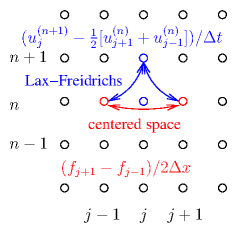Figure 7.5: Forward derivative in Time ($n$) but from the mean of the adjacent points and Centered in Space ($j$) is the Lax Friedrichs finite difference scheme, which is stable provided $\mathit{\Delta }t\le \mathit{\Delta }x/|\mathit{\lambda }|$.
This is then called the Lax-Friedrichs method.
 ${\mathbf{u}}_{j}^{\left(n+1\right)}-\left({\mathbf{u}}_{j-1}^{\left(n\right)}+{\mathbf{u}}_{j+1}^{\left(n\right)}\right)/2=-\frac{\mathit{\Delta }t}{2\mathit{\Delta }x}\left({\mathbf{f}}_{j+1}^{\left(n\right)}-{\mathbf{f}}_{j-1}^{\left(n\right)}\right)=-\frac{\mathit{\Delta }t}{2\mathit{\Delta }x}\mathbf{J}\left({\mathbf{u}}_{j+1}^{\left(n\right)}-{\mathbf{u}}_{j-1}^{\left(n\right)}\right).$ $\left(7.27\right)$
The student should verify that replacing $\mathbf{J}$ with $\mathit{\lambda }$ for a scalar version of these equations, the resulting amplification factor is
 $A=\mathrm{cos}\left({k}_{x}\mathit{\Delta }x\right)-i\frac{\mathit{\Delta }t\mathit{\lambda }}{\mathit{\Delta }x}\mathrm{sin}\left({k}_{x}\mathit{\Delta }x\right).$ $\left(7.28\right)$
As ${k}_{x}$ varies, this is the equation of an ellipse in the complex plane. For stability, this ellipse must be entirely inside the unit circle, which requires the imaginary coefficient's magnitude to be less than or equal to 1
 $\mathit{\Delta }t\le \mathit{\Delta }x/|\mathit{\lambda }|.$ $\left(7.29\right)$
For our fluid example this is $\mathit{\Delta }t\le \mathit{\Delta }x/|v±{c}_{s}|$. Equation (7.29) is called the Courant-Friedrichs-Lewy (CFL) condition. It applies to essentially all explicit schemes for hyperbolic equations. It says that $\mathit{\Delta }t$ must be less than the time it takes for influence to propagate at the characteristic speed(s) (given by the eigenvalues of $\mathbf{J}$) from the prior adjacent nodes. If it were greater, then influence from other nodes, not taken into account in the difference scheme, would influence the solution.

### 7.3.3  Lax-Wendroff achieves second order accuracy

The low order errors of the Lax-Friedrichs scheme make it of little practical value. It has a substantial level of spurious numerical diffusion that damps out perturbations that should not be damped. For example the simple fluid we've used to illustrate the issues has no physical dissipation, yet for some modes Lax-Friedrichs gives $|A|$ substantially less than one. They are damped. A better scheme, which is second order in time and still stable, is the Lax-Wendroff scheme. The advance is implemented in two steps:
 ${\mathbf{u}}_{j+1/2}^{\left(n+1/2\right)}=\frac{1}{2}\left({\mathbf{u}}_{j+1}^{\left(n\right)}+{\mathbf{u}}_{j}^{\left(n\right)}\right)-\frac{\mathit{\Delta }t}{2\mathit{\Delta }x}\left({\mathbf{f}}_{j+1}^{\left(n\right)}-{\mathbf{f}}_{j}^{\left(n\right)}\right)$ $\left(7.30\right)$

 ${\mathbf{u}}_{j}^{\left(n+1\right)}={\mathbf{u}}_{j}^{\left(n\right)}-\frac{\mathit{\Delta }t}{\mathit{\Delta }x}\left({\mathbf{f}}_{j+1/2}^{\left(n+1/2\right)}-{\mathbf{f}}_{j-1/2}^{\left(n+1/2\right)}\right).$ $\left(7.31\right)$
Fig. 7.6 shows the schematic.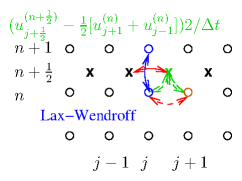Figure 7.6: The Lax-Wendroff two-step scheme first (dashed lines) generates $u$ and hence $f$ values at the half-time-step $n+\frac{1}{2}$, by a Lax-Friedrichs advance to (X). Then it uses a centered time, centered space full step advance based upon ${\mathbf{f}}^{\left(n+1/2\right)}$, from the ${\mathbf{u}}^{\left(n\right)}$.
The first step is like a Lax-Friedrichs half-step to the half-way positions. Then the fluxes are evaluated again, at the half-step times and positions using the ${\mathbf{u}}^{\left(n+1/2\right)}$ values, to find the ${\mathbf{f}}^{\left(n+1/2\right)}$. Those are used in the second step to advance all the way from $n$ to $n+1$ in a properly centered manner. The amplification factor for the combined step can be shown to be
 $A=1-i\frac{\mathit{\Delta }t\mathit{\lambda }}{\mathit{\Delta }x}\mathrm{sin}\left({k}_{x}\mathit{\Delta }x\right)+{\left(\frac{\mathit{\Delta }t\mathit{\lambda }}{\mathit{\Delta }x}\right)}^{2}\left[\mathrm{cos}\left({k}_{x}\mathit{\Delta }x\right)-1\right]$ $\left(7.32\right)$
which gives stability if $\frac{\mathit{\Delta }t|\mathit{\lambda }|}{\mathit{\Delta }x}\le 1$: the CFL condition, the same as before.
There are several other schemes in regular use for solving first order hyperbolic problems to second order accuracy. They practically all use multi-step approaches like the Lax-Wendroff method.

## Worked Example. Stability of Lax-Wendroff scheme.

Derive the amplification factor for the Lax-Wendroff scheme and verify the stability condition $\frac{\mathit{\Delta }t|\mathit{\lambda }|}{\mathit{\Delta }x}\le 1$.

Start with the formula for the first time half-step: eq. (7.30). For stability analysis (but not in implementing an actual numerical scheme), approximate the Jacobian matrix locally as uniform, and substitute $\mathbf{f}=\mathbf{J}\mathbf{u}$ at all the required mesh positions, deriving
 $\begin{array}{cc}\hfill {\mathbf{u}}_{j+1/2}^{\left(n+1/2\right)}& =\frac{1}{2}\left({\mathbf{u}}_{j+1}^{\left(n\right)}+{\mathbf{u}}_{j}^{\left(n\right)}\right)-\frac{\mathit{\Delta }t}{2\mathit{\Delta }x}\mathbf{J}\left({\mathbf{u}}_{j+1}^{\left(n\right)}-{\mathbf{u}}_{j}^{\left(n\right)}\right)\hfill \\ \hfill & =\frac{1}{2}\left[\left(\mathbf{I}-\frac{\mathit{\Delta }t}{\mathit{\Delta }x}\mathbf{J}\right){\mathbf{u}}_{j+1}^{\left(n\right)}+\left(\mathbf{I}+\frac{\mathit{\Delta }t}{\mathit{\Delta }x}\mathbf{J}\right){\mathbf{u}}_{j}^{\left(n\right)}\right].\hfill \end{array}$ $\left(7.33\right)$
Similarly, the second half-step can be written:
 ${\mathbf{u}}_{j}^{\left(n+1\right)}={\mathbf{u}}_{j}^{\left(n\right)}-\frac{\mathit{\Delta }t}{\mathit{\Delta }x}\mathbf{J}\left({\mathbf{u}}_{j+1/2}^{\left(n+1/2\right)}-{\mathbf{u}}_{j-1/2}^{\left(n+1/2\right)}\right).$ $\left(7.34\right)$
Substitute for the half-step values from eq. (7.33) to find:
 $\begin{array}{ccc}\multicolumn{1}{c}{{\mathbf{u}}_{j}^{\left(n+1\right)}-{\mathbf{u}}_{j}^{\left(n\right)}}& =\hfill & -\frac{\mathit{\Delta }t}{2\mathit{\Delta }x}\mathbf{J}\left[\left(\mathbf{I}-\frac{\mathit{\Delta }t}{\mathit{\Delta }x}\mathbf{J}\right){\mathbf{u}}_{j+1}^{\left(n\right)}+\left(\mathbf{I}+\frac{\mathit{\Delta }t}{\mathit{\Delta }x}\mathbf{J}\right){\mathbf{u}}_{j}^{\left(n\right)}\hfill \\ \multicolumn{1}{c}{}& \hfill & \mathrm{ }\mathrm{ }-\left(\mathbf{I}-\frac{\mathit{\Delta }t}{\mathit{\Delta }x}\mathbf{J}\right){\mathbf{u}}_{j}^{\left(n\right)}-\left(\mathbf{I}+\frac{\mathit{\Delta }t}{\mathit{\Delta }x}\mathbf{J}\right){\mathbf{u}}_{j-1}^{\left(n\right)}\right]\hfill & \hfill \left(7.35\right)\\ \multicolumn{1}{c}{}& =\hfill & -\frac{\mathit{\Delta }t}{2\mathit{\Delta }x}\mathbf{J}\left[\left({\mathbf{u}}_{j+1}^{\left(n\right)}-{\mathbf{u}}_{j-1}^{\left(n\right)}\right)-\frac{\mathit{\Delta }t}{\mathit{\Delta }x}\mathbf{J}\left({\mathbf{u}}_{j+1}^{\left(n\right)}-2{\mathbf{u}}_{j}^{\left(n\right)}+{\mathbf{u}}_{j-1}^{\left(n\right)}\right)\right].\hfill \end{array}$

Now we consider an eigenmode of $\mathbf{J}$, so we can substitute the eigenvalue $\mathit{\lambda }$ for $\mathbf{J}$, everywhere in the above expression. And we consider a spatial Fourier mode, for which ${\mathbf{u}}_{j}\propto {e}^{{\mathit{ik}}_{x}j\mathit{\Delta }x}$. The equation can then be written
 ${\mathbf{u}}_{j}^{\left(n+1\right)}-{\mathbf{u}}_{j}^{\left(n\right)}=-\frac{\mathit{\Delta }t}{2\mathit{\Delta }x}\mathit{\lambda }\left[\left({e}^{{\mathit{ik}}_{x}\mathit{\Delta }x}-{e}^{-{\mathit{ik}}_{x}\mathit{\Delta }x}\right)+\frac{\mathit{\Delta }t}{\mathit{\Delta }x}\mathit{\lambda }\left({e}^{{\mathit{ik}}_{x}\mathit{\Delta }x}-2+{e}^{-{\mathit{ik}}_{x}\mathit{\Delta }x}\right)\right]{\mathbf{u}}_{j}^{\left(n\right)},$ $\left(7.36\right)$
or in other words
 ${\mathbf{u}}_{j}^{\left(n+1\right)}=\left\{1-\frac{\mathit{\Delta }t\mathit{\lambda }}{\mathit{\Delta }x}i\mathrm{sin}\left({k}_{x}\mathit{\Delta }x\right)+{\left(\frac{\mathit{\Delta }t\mathit{\lambda }}{\mathit{\Delta }x}\right)}^{2}\left[\mathrm{cos}\left({k}_{x}\mathit{\Delta }x\right)-1\right]\right\}{\mathbf{u}}_{j}^{\left(n\right)}.$ $\left(7.37\right)$
The coefficient of ${\mathbf{u}}_{j}^{\left(n\right)}$ is the amplification factor, $A$. Its squared absolute value is
 $\begin{array}{ccc}\multicolumn{1}{c}{|A{|}^{2}}& =\hfill & {\left\{1+{\left(\frac{\mathit{\Delta }t\mathit{\lambda }}{\mathit{\Delta }x}\right)}^{2}\left[\mathrm{cos}\left({k}_{x}\mathit{\Delta }x\right)-1\right]\right\}}^{2}+{\left\{\frac{\mathit{\Delta }t\mathit{\lambda }}{\mathit{\Delta }x}\mathrm{sin}\left({k}_{x}\mathit{\Delta }x\right)\right\}}^{2}\hfill \\ \multicolumn{1}{c}{}& =\hfill & 1+{\left(\frac{\mathit{\Delta }t\mathit{\lambda }}{\mathit{\Delta }x}\right)}^{2}\left[2\mathrm{cos}\left({k}_{x}\mathit{\Delta }x\right)-2+{\mathrm{sin}}^{2}\left({k}_{x}\mathit{\Delta }x\right)\right]\hfill \\ \multicolumn{1}{c}{}& \hfill & \mathrm{ }+{\left(\frac{\mathit{\Delta }t\mathit{\lambda }}{\mathit{\Delta }x}\right)}^{4}\left[\mathrm{cos}\left({k}_{x}\mathit{\Delta }x\right)-1{\right]}^{2}\hfill & \hfill \left(7.38\right)\\ \multicolumn{1}{c}{}& =\hfill & 1+\left[-{\left(\frac{\mathit{\Delta }t\mathit{\lambda }}{\mathit{\Delta }x}\right)}^{2}+{\left(\frac{\mathit{\Delta }t\mathit{\lambda }}{\mathit{\Delta }x}\right)}^{4}\right]\left[\mathrm{cos}\left({k}_{x}\mathit{\Delta }x\right)-1{\right]}^{2}.\hfill \end{array}$

Thus $|A{|}^{2}\le 1$ provided $\frac{\mathit{\Delta }t|\mathit{\lambda }|}{\mathit{\Delta }x}\le 1$, which is the stability criterion.

## 7.4  Worked Example: Three-dimensional fluids

Formulate a finite difference representation of the hyperbolic equations for a source-free, inviscid, isotropic fluid in three-dimensions plus time, when the equation of state is $p=p\left(\mathit{\rho }\right)$. Assume the eigenvalue of the Jacobian of the linearized system (perturbation propagation speed) is known, $\mathit{\lambda }$, and that the eigenmode is longitudinal; deduce the condition governing the stable explicit timestep for centered spatial differences on a uniform cartesian grid spaced unequally in the different axis directions.

We use the density $\mathit{\rho }$ and the flux density $\mathbit{\Gamma }$ as the elements of the state vector $\mathbf{u}$. In 3-dimensions, a vector quantity like $\mathbit{\Gamma }$ has three components, ${\mathit{\Gamma }}_{\mathit{\alpha }}, \mathit{\alpha }=1,2,3$. So the state vector has a total of four.
 $\mathbf{u}=\left(\begin{array}{c}\hfill \mathit{\rho }\hfill \\ \hfill \mathbit{\Gamma }\hfill \end{array}\right)=\left(\begin{array}{c}\hfill \mathit{\rho }\hfill \\ \hfill {\mathit{\Gamma }}_{1}\hfill \\ \hfill {\mathit{\Gamma }}_{2}\hfill \\ \hfill {\mathit{\Gamma }}_{3}\hfill \end{array}\right)$ $\left(7.39\right)$
The continuity () and momentum (7.4) equations are written with the time and space differentials separated on the left and the right hand sides, and we replace $\mathit{\rho }\mathbit{v}$ everywhere with $\mathbit{\Gamma }$.
 $\frac{\partial \mathit{\rho }}{\partial t}=-\nabla .\left(\mathit{\rho }\mathbit{v}\right)=-\nabla .\mathbit{\Gamma },$ $\left(7.40\right)$

 $\frac{\partial \mathbit{\Gamma }}{\partial t}=-\nabla .\left(\mathit{\rho }\mathbit{v}\mathbit{v}\right)-\nabla p=-\nabla .\left(\mathbit{\Gamma }\mathbit{\Gamma }/\mathit{\rho }+\mathbf{I}p\right).$ $\left(7.41\right)$
In 3-dimensions, these are four scalar equations in total. The combined state-space form is
 $\frac{\partial \mathbf{u}}{\partial t}=-\nabla .\mathbf{f},$ $\left(7.42\right)$
where $\nabla .=\sum _{\mathit{\alpha }}{\stackrel{^}{\mathbit{x}}}_{\mathit{\alpha }}.\frac{\partial }{\partial {x}_{\mathit{\alpha }}}$, the spatial-3-vector divergence, operates separately on each of the four (3-vector) entries of the state-space column-vector
 $\mathbf{f}=\left(\begin{array}{c}\hfill \mathbit{\Gamma }\hfill \\ \hfill \mathbit{\Gamma }{\mathit{\Gamma }}_{1}/\mathit{\rho }+p{\stackrel{^}{\mathbit{x}}}_{1}\hfill \\ \hfill \mathbit{\Gamma }{\mathit{\Gamma }}_{2}/\mathit{\rho }+p{\stackrel{^}{\mathbit{x}}}_{2}\hfill \\ \hfill \mathbit{\Gamma }{\mathit{\Gamma }}_{3}/\mathit{\rho }+p{\stackrel{^}{\mathbit{x}}}_{3}\hfill \end{array}\right)=\left(\begin{array}{c}\hfill \mathbit{\Gamma }\hfill \\ \hfill \mathbit{\Gamma }\mathbit{\Gamma }/\mathit{\rho }+p\mathbf{I}\hfill \end{array}\right).$ $\left(7.43\right)$
The spatial discrete difference scheme may be written in terms of three cartesian indices $i$, $j$, $k$, of the mesh, as
 $\begin{array}{ccc}\multicolumn{1}{c}{\nabla .\mathbf{f}}& =\hfill & \frac{{\stackrel{^}{\mathbit{x}}}_{1}}{2\mathit{\Delta }{x}_{1}}.\left({\mathbf{f}}_{\left(i+1\right)\mathit{jk}}^{\left(n\right)}-{\mathbf{f}}_{\left(i-1\right)\mathit{jk}}^{\left(n\right)}\right)+\frac{{\stackrel{^}{\mathbit{x}}}_{2}}{2\mathit{\Delta }{x}_{2}}.\left({\mathbf{f}}_{i\left(j+1\right)k}^{\left(n\right)}-{\mathbf{f}}_{i\left(j-1\right)k}^{\left(n\right)}\right)\hfill \\ \multicolumn{1}{c}{}& \hfill & \mathrm{ }\mathrm{ }+\frac{{\stackrel{^}{\mathbit{x}}}_{3}}{2\mathit{\Delta }{x}_{3}}.\left({\mathbf{f}}_{\mathit{ij}\left(k+1\right)}^{\left(n\right)}-{\mathbf{f}}_{\mathit{ij}\left(k-1\right)}^{\left(n\right)}\right).\hfill & \hfill \left(7.44\right)\end{array}$

We are told that the eigenvalue of the state system is $\mathit{\lambda }$ and the eigenmode is longitudinal${}^{44}$. Therefore, for a plane wave proportional to $\mathrm{exp}\left(i\mathbit{k}.\mathbit{x}\right)$ that is an eigenmode of the state, each state-component of $\mathbf{f}$ is oriented in the spatial-direction $\mathbit{k}$. Write the unit vector $\stackrel{^}{\mathbit{k}}=\left({\stackrel{^}{k}}_{1},{\stackrel{^}{k}}_{3},{\stackrel{^}{k}}_{3}\right)$, and $\mathbit{k}=k\stackrel{^}{\mathbit{k}}$. Then for this plane wave we can replace each ${\stackrel{^}{\mathbit{x}}}_{\mathit{\alpha }}.\mathbf{f}$ with $\mathit{\lambda }{\stackrel{^}{k}}_{\mathit{\alpha }}\mathbf{u}$ to obtain

 $\nabla .\mathbf{f}=\frac{1}{2}\left[\frac{\mathit{\lambda }{\stackrel{^}{k}}_{1}}{\mathit{\Delta }{x}_{1}}\left({\mathbf{u}}_{\left(i+1\right)\mathit{jk}}^{\left(n\right)}-{\mathbf{u}}_{\left(i-1\right)\mathit{jk}}^{\left(n\right)}\right)-\frac{\mathit{\lambda }{\stackrel{^}{k}}_{2}}{\mathit{\Delta }{x}_{2}}\left({\mathbf{u}}_{i\left(j+1\right)k}^{\left(n\right)}-{\mathbf{u}}_{i\left(j-1\right)k}^{\left(n\right)}\right)-\frac{\mathit{\lambda }{\stackrel{^}{k}}_{3}}{\mathit{\Delta }{x}_{3}}\left({\mathbf{u}}_{\mathit{ij}\left(k+1\right)}^{\left(n\right)}-{\mathbf{u}}_{\mathit{ij}\left(k-1\right)}^{\left(n\right)}\right)\right].$ $\left(7.45\right)$
Substituting for the variation of $\mathbf{u}$ with spatial index, e.g. $\left({\mathbf{u}}_{\mathit{ij}\left(k+1\right)}^{\left(n\right)}-{\mathbf{u}}_{\mathit{ij}\left(k-1\right)}^{\left(n\right)}\right)$ $=\mathrm{exp}\left({\mathit{ik}}_{3}\mathit{\Delta }{x}_{3}\right)-\mathrm{exp}\left(-{\mathit{ik}}_{3}\mathit{\Delta }{x}_{3}\right)=2i\mathrm{sin}\left({k}_{3}\mathit{\Delta }{x}_{3}\right)$, this form reduces the finite difference equations to
 ${\mathbf{u}}_{\mathit{ijk}}^{\left(n+1\right)}-{\mathbf{u}}_{s}^{\left(n\right)}=-\mathit{\Delta }t\nabla .\mathbf{f}=-\sum _{\mathit{\alpha }}\frac{i\mathit{\Delta }t\mathit{\lambda }{\stackrel{^}{k}}_{\mathit{\alpha }}}{\mathit{\Delta }{x}_{\mathit{\alpha }}}\mathrm{sin}\left(k{\stackrel{^}{k}}_{\mathit{\alpha }}\mathit{\Delta }{x}_{\mathit{\alpha }}\right){\mathbf{u}}_{\mathit{ijk}},$ $\left(7.46\right)$
where ${\mathbf{u}}_{s}^{\left(n\right)}$ denotes the mesh expression used for the current time $\left(n\right)$. For example, a Lax-Friedrichs choice ${\mathbf{u}}_{s}^{\left(n\right)}=\frac{1}{6}\left({\mathbf{u}}_{\left(i-1\right)\mathit{jk}}^{\left(n\right)}+{\mathbf{u}}_{\left(i+1\right)\mathit{jk}}^{\left(n\right)}+{\mathbf{u}}_{i\left(j-1\right)k}^{\left(n\right)}+{\mathbf{u}}_{i\left(j+1\right)k}^{\left(n\right)}+{\mathbf{u}}_{\mathit{ij}\left(k-1\right)}^{\left(n\right)}+{\mathbf{u}}_{\mathit{ij}\left(k+1\right)}^{\left(n\right)}\right)$ leads to an amplification factor
 $A=\sum _{\mathit{\alpha }}\left[\frac{1}{3}\mathrm{cos}\left(k{\stackrel{^}{k}}_{\mathit{\alpha }}\mathit{\Delta }{x}_{\mathit{\alpha }}\right)-\frac{i\mathit{\Delta }t\mathit{\lambda }{\stackrel{^}{k}}_{\mathit{\alpha }}}{\mathit{\Delta }{x}_{\mathit{\alpha }}}\mathrm{sin}\left(k{\stackrel{^}{k}}_{\mathit{\alpha }}\mathit{\Delta }{x}_{\mathit{\alpha }}\right)\right].$ $\left(7.47\right)$
We require $|A{|}^{2}\le 1$ for all modes to avoid instability. The worst case for stability occurs when all ${\stackrel{^}{k}}_{\mathit{\alpha }}\mathit{\Delta }{x}_{\mathit{\alpha }}$ have the same value, which we'll denote $\mathit{\Delta }=\left(\sum _{\mathit{\alpha }}1/\mathit{\Delta }{x}_{\mathit{\alpha }}^{2}{\right)}^{-1/2}$. Avoiding instability in this case requires that
 $\mathit{\Delta }t|\mathit{\lambda }|\sum _{\mathit{\alpha }}\frac{{\stackrel{^}{k}}_{\mathit{\alpha }}^{2}}{{\stackrel{^}{k}}_{\mathit{\alpha }}\mathit{\Delta }{x}_{\mathit{\alpha }}}=\frac{\mathit{\Delta }t|\mathit{\lambda }|}{\mathit{\Delta }}\le 1.$ $\left(7.48\right)$
Notice, by considering $k\mathit{\Delta }=\mathit{\pi }/2$, that the criterion must be satisfied for stability, regardless of the precise form chosen for ${\mathbf{u}}_{s}$, so long as that form is symmetric in each coordinate direction, and hence gives rise to a real contribution to $A$. The criterion is thus universally necessary for any symmetric centered spatial difference scheme, when time differences are explicit, but it is not always sufficient.
When all the $\mathit{\Delta }{x}_{\mathit{\alpha }}$ are equal, then $\mathit{\Delta }=\mathit{\Delta }x/\sqrt{3}$, and the CFL condition for stability when $v$ is small (so $\mathit{\lambda }={c}_{s}$) is $\mathit{\Delta }t\le \frac{\mathit{\Delta }x}{{c}_{s}\sqrt{3}}$. If, by contrast, for some direction $\mathit{\beta }$, $\mathit{\Delta }{x}_{\mathit{\beta }}$ is much smaller than the other two grid spacings, then $\mathit{\Delta }\approx \mathit{\Delta }{x}_{\mathit{\beta }}$ and stability requires $\mathit{\Delta }t\le \frac{\mathit{\Delta }{x}_{\mathit{\beta }}}{{c}_{s}}$.

## Exercise 7. Fluids and Hyperbolic Equations.

1. Prove equation 7.29, the amplification factor for the Lax Friedrichs scheme.

2. Consider an isothermal gas in one dimension. It obeys the equations
 $\begin{array}{cccc}\mathit{Continuity}:\mathrm{ }\hfill & \hfill \frac{\partial \mathit{\rho }}{\partial t}+\frac{\partial }{\partial x}\left(\mathit{\rho }v\right)& \hfill =\hfill & 0\hfill \\ \mathit{Momentum}:\mathrm{ }\hfill & \hfill \frac{\partial }{\partial t}\left(\mathit{\rho }v\right)+\frac{\partial }{\partial x}\left(\mathit{\rho }{v}^{2}\right)& \hfill =\hfill & -\frac{\partial }{\partial x}p\hfill \\ \mathit{State}:\mathrm{ }\hfill & \hfill p& \hfill =\hfill & \mathit{\rho }\left(\mathit{kT}/m\right)\hfill \end{array}$

with $\mathit{kT}/m$ simply a constant equal to the ratio of the temperature in energy units to the gas molecule mass $m$.
(a) Convert this into the form of a state and flux vector equation
 $\frac{\partial \mathbf{u}}{\partial t}=-\frac{\partial \mathbf{f}}{\partial x}.$

where
 $\mathbf{u}=\left(\begin{array}{c}\hfill \mathit{\rho }\hfill \\ \hfill \mathit{\Gamma }\hfill \end{array}\right)$

is the state vector ($\mathit{\Gamma }=\mathit{\rho }v$) and you should give the flux vector $\mathbf{f}$.
(b) Calculate the Jacobian matrix $\mathbf{J}=\partial \mathbf{f}/\partial \mathbf{u}$.
(c) Find its eigenvalues.

3. Find the finite difference form and CFL stability when the linearized eigenmode is longitudinal with eigenvalue $\mathit{\lambda }$, for the Lax-Wendroff scheme in two space-dimensions.